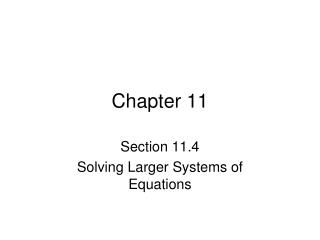DownloadDownload PresentationChapter 11

# Chapter 11

Download Presentation## Chapter 11

- - - - - - - - - - - - - - - - - - - - - - - - - - - E N D - - - - - - - - - - - - - - - - - - - - - - - - - - -
##### Presentation Transcript

1. Chapter 11 Section 11.4 Solving Larger Systems of Equations

2. Larger Systems of Equations A system of equations that has 3 or more variables can be solved by combining both the elimination method and the substitution method. 1. You eliminate variables from the other equations until you get down to one equation with one variable. 2. Solve that one equation for the variable and substitute it back into the other equations and solve for the one variable that remains. Example Solve the System of Equations: Multiply Equation 1 by ¼. Solve Equation 3 for z. Substitute y and z into Equation 1. Multiply Equation 1 by -2 and add to Equation 2. Multiply Equation 1 by -3 and add to Equation 3. Solve Equation 2 for y. The solution is: x = 3, y = 2, z = -3

3. Systems of Equations and Matrices Systems of equations can be represented with matrices in a certain way. 1. Each row corresponds to an equation. 2. Each column to a variable and the last column to the constants. We write the variables on one side of the equation and the constants on the other. In the matrix separate the variables from the constants with a line (sometimes dashed). The entries of the matrix are the coefficients of the variables. It is important that if a variable does not show up in an equation that means the coefficient is 0 and that entry in the matrix is 0. The entries on the other side of the line are the constants. system of equations system of equations Matrix Matrix Sometimes algebra might be needed to change the equations to a matrix.

4. Subscripted Variables In systems of equations where more than 3 variables (sometime for 2 and 3 variables) are needed instead of using regular variable we use just one x but put subscripts on it xi "read x sub i". Matrices with 1's and 0's A matrix that has 1's down the diagonal from top left to bottom right is easy to read the simultaneous solution of system of equations right from the matrix. They are the entries in the constants column. Matrix Equations Simultaneous Solution

5. Row Operations We will use specific row operations to change a matrix from the original equation form to the type with 1's down the diagonal and 0's everywhere else. The row operation we use keep the simultaneous solution ROW OPERATIONS For a matrix A there are 4 row operations that are allowed. The way you refer to each row operation below the second way is how the TI-83 graphing calculator refers to them. Ri RjRowSwap([A],i,j) Interchange the ith and jth rows. Ri+Rj Row+([A],i,j) Add the ith row to the jth row. mRi *Row(m,[A],i) Multiply the ith row by the number m. mRi+Rj *Row+(m,[A],i,j) Multiply the ith row by the number m and add it to the jth row.

6. Examples of row operations. RowSwap Row+ R1R3 RowSwap([A],1,3) R1+R3 Row+([A],1,3) *Row *Row+ ¼R1 *Row(¼,[A],1) -2R1+R3 *Row+(-2,[A],1,3)

7. Pivoting The process of using elementary row operations to "clear out" a column and get a 1 in the diagonal position in the column and then zeros in all others is called pivoting. Only the row operations are allowed when doing this process. The diagonal entries are the pivot positions. Pivot Position -⅓R2 *Row(-⅓,[Ans],2) ½R1 *Row(½,[A],1) -3R1+R2 *Row+(-3,[Ans],1,2) -4R2+R1 *Row+(-4,[Ans],2,1) The second column is "cleared out"! The first column is "cleared out"!

8. Gauss-Jordan Elimination Gauss-Jordan Elimination is a process of pivoting column by column until you have all but the last column "cleared". R1R2 RowSwap([A],1,2) ½R1 *Row(½,[Ans],1) R1R2 RowSwap([A],1,2) -3R2+R1 *Row+(-3,[Ans],2,1) -2R2+R3 *Row+(-2,[Ans],2,3) ¼R1 *Row(¼,[Ans],1) ½R3 *Row(½,[Ans],3) -½R2 *Row(-½,[Ans],2) 13R3+R1 *Row+(13,[Ans],3,1) -5R3+R2 *Row+(-5,[Ans],3,2) -½R2+R1 *Row+(-½,[Ans],2,1)

9. Putting it Together Lets use matrix simplification (i.e. Gauss-Jordan Elimination) to solve the system of equations below. Converting back to equations gives: ⅓R1 *Row(⅓,[A],1) -2R1+R2 *Row+(-2,[Ans],1,2) We form the matrix. 3R2 *Row(3,[Ans],2) Check: -7/3R2+R1 *Row+(- 7/3,[Ans],2,1) Call this A and simplify it